# c++类的类型转换函数

#include <stdio.h>

class TestCls{
public:
int a;

TestCls(int x = 0) : a(x)
{}
};

int main(void)
{
TestCls t1;

int i = t1;

printf("i = %d\n", i);

return 0;
}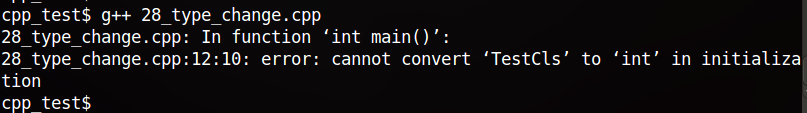int i = (int)t1;

operator Type()
{
Type ret;

//...

return ret;
}

class TestCls{
public:
int a;

TestCls(int x = 5) : a(x)
{}

/* 类型转换函数，将TestCls类转换为int类型 */
operator int()
{
return a;
}

};

int main(void)
{
TestCls t1;

int i = t1;

printf("i = %d\n", i);

return 0;
}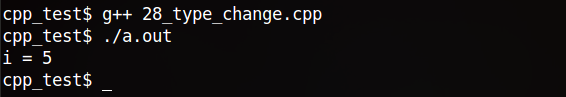1. 类型转换函数必须是成员函数，不能指定其返回类型，并且形参必须为空，返回值是隐含的，返回值类型是和转换的类型Type是相同的，在本例子中为int。
2. Type表示内置类型名、类类型名或者是类型别名(typedef)。除了void外，任何可作为函数返回类型的类型都可以定义转换函数的目标转换类型。一般不允许转换为数组或函数类型，但是可以转换为指针类型以及引用类型
3. 类型转换函数一般不应该改变被转换的对象，因此转换函数通常属性被定义为const。

int i = t1;能够编译运行，因为调用了类型转换t1对象的类型转换函数operator int()。


class A
{
private:
int a;

public:
A(int x = 0) : a(x)
{}

char value()
{
return a;
}
};

class B
{
private:
char c;

public:
B(char y = 0) : c(y)
{}

char value()
{
return c;
}
};

int main(void)
{
A class_a;
B class_b(100);

class_a = class_b;

printf("class_a.a = %d\n", class_a.value());

return 0;
}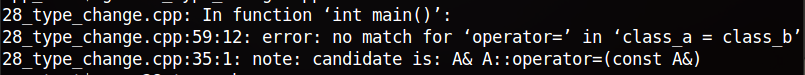B类定义类型转换函数后：

class B
{
private:
char c;

public:
B(char y = 0) : c(y)
{}

char value()
{
return a;
}

operator A ()
{
A tmp(c);
return tmp;
}
};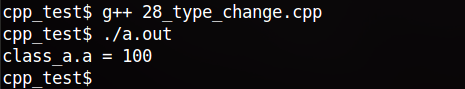class_a = class_b;其实等价于class_a = class_b.operator int();


class B;        //前向声明

class A
{
private:
int a;

public:
A(int x = 0) : a(x)
{}

A(B& cB)
{
}

char value()
{
return a;
}
};

class B
{
public:
char c;

public:
B(char y = 0) : c(y)
{}

char value()
{
return c;
}

/*operator A ()
{
A tmp(c);
return tmp;
}*/
};

int main(void)
{
A class_a;
B class_b(100);

class_a = class_b;

return 0;
}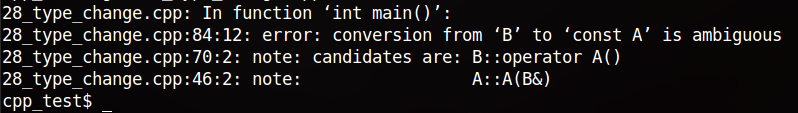class B
{
public:
char c;

public:
B(char y = 0) : c(y)
{}

char value()
{
return c;
}

A toA ()
{
A tmp(c);
return tmp;
}
};

int main(void)
{
A class_a;
B class_b(100);

class_a = class_b.toA();

printf("class_a.a = %d\n", class_a.value());

return 0;
}

02-13
05-23173
02-281200
08-201564
08-10213
01-057348
03-19280
06-0440
03-31311
05-1768
08-10822
02-26444
06-293917
08-28295
11-181825
04-172431
03-27786

### “相关推荐”对你有帮助么？

•非常没帮助
•没帮助
•一般
•有帮助
•非常有帮助

©️2022 CSDN 皮肤主题：编程工作室 设计师：CSDN官方博客被折叠的  条评论 为什么被折叠?到【灌水乐园】发言mybright_

¥2 ¥4 ¥6 ¥10 ¥20余额支付 (余额：-- )扫码支付获取中扫码支付点击重新获取扫码支付1.余额是钱包充值的虚拟货币，按照1:1的比例进行支付金额的抵扣。
2.余额无法直接购买下载，可以购买VIP、C币套餐、付费专栏及课程。余额充值